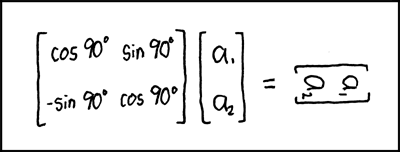# Twisting one corner?

#### cubemaster13

##### Member
Twisting one corner? (FAKE)

I am sure I am not the only one to see this but MeMyselfAndPi uploaded a video of him twisting one corner on a 3x3 cube. I tried it for myself and I was completely amazed to see that it actually worked. But how is this possible? Someone use math and smart stuff to explain this to me!!!!

Here is the link by the way: http://www.youtube.com/watch?v=1N0reAJmeI0&feature=feedu

I just realized that he is approving comments for the video so something is probably up with this. But I am almost positive this worked for me before...

This is fake... You can kinda sorta here or see a cut a 51 seconds in... He puts a lot of effort to turn the cube around.

Last edited:

#### Cool Frog

##### Member
So now there are 2x43 quintillion possible cases on the 3x3 (Excuse my completely terrible mathematical skills.)

#### cmhardw

##### Premium Member
But how is this possible? Someone use math and smart stuff to explain this to me!!!!
He was probably using:
$$\frac{3 \sin{\left(\frac{2\pi x}{3}\right)}}{x}$$

to model the rotations of the corner pieces. Notice that the numerator has period 3 for the three corner twists.

My guess is that the algs most people use do something along the lines of:

$$\int_{-1}^{1} \frac{3 \sin{\left(\frac{2\pi x}{3}\right)}}{x}\,dx$$

which obviously doesn't work since you're integrating over a discontinuity. So clearly, what MeMyselfandPi probably figured out was the very clever:

$$2 \left[ \lim_{a \to 0^{+}} \int_{a}^{1} \frac{3 \sin{\left(\frac{2\pi x}{3}\right)}}{x}\,dx \right]$$

This would clearly avoid the integration over a discontinuity, and thus avoid the impossibility of the single corner twist!

Very cool!Last edited:

#### wontolla

##### Member
Somebody let Lars Petrus know about this. He once had problems with the "twisted corner".

#### Specs112

##### Member
He was probably using:
$$\frac{3 \sin{\left(\frac{2\pi x}{3}\right)}}{x}$$

to model the rotations of the corner pieces. Notice that the numerator has period 3 for the three corner twists.

My guess is that the algs most people use do something along the lines of:

$$\int_{-1}^{1} \frac{3 \sin{\left(\frac{2\pi x}{3}\right)}}{x}\,dx$$

which obviously doesn't work since you're integrating over a discontinuity. So clearly, what MeMyselfandPi probably figured out was the very clever:

$$2 \left[ \lim_{a \to 0^{+}} \int_{a}^{1} \frac{3 \sin{\left(\frac{2\pi x}{3}\right)}}{x}\,dx \right]$$

This would clearly avoid the integration over a discontinuity, and thus avoid the impossibility of the single corner twist!

Very cool!I'm not familiar with that math, does it work something like this?#### qqwref

##### Member
Wow, you read xkcd - you must be really clever.

I liked this video. The trickery was non-obvious enough that it made me go "wait a minute". Reminds me or Kir's secret parity video.

#### Zubon

##### Member
That D2 has a very suspicious click....

But this is a cool trick.#### cmhardw

##### Premium Member
I'm not familiar with that math, does it work something like this?No, not really. That is a cool picture thoughThe math in my post shall be explained in the spoiler below:
Actually, the math in my post does not relate to cubing in the slightest. I was simply responding to this:
Someone use math and smart stuff to explain this to me!!!!
Since it is impossible to twist one corner on a Rubik's cube by simply turning the faces, then asking someone to explain an impossible outcome using math effectively divides by zero and allows you to do whatever you want for the fun of itI simply took the opportunity and ran with it#### Owen

##### Member
Yeah, it's April Fools. MM&P is getting better at this. Last year's April fools was hilarious!

#### Cubenovice

##### Forever Slow
Don't care about the corner but where is the challenge if you have colors on an arrow cube?

#### uberCuber

##### Member
He was probably using:
$$\frac{3 \sin{\left(\frac{2\pi x}{3}\right)}}{x}$$

to model the rotations of the corner pieces. Notice that the numerator has period 3 for the three corner twists.

My guess is that the algs most people use do something along the lines of:

$$\int_{-1}^{1} \frac{3 \sin{\left(\frac{2\pi x}{3}\right)}}{x}\,dx$$

which obviously doesn't work since you're integrating over a discontinuity. So clearly, what MeMyselfandPi probably figured out was the very clever:

$$2 \left[ \lim_{a \to 0^{+}} \int_{a}^{1} \frac{3 \sin{\left(\frac{2\pi x}{3}\right)}}{x}\,dx \right]$$

This would clearly avoid the integration over a discontinuity, and thus avoid the impossibility of the single corner twist!

Very cool!That is genius, I never thought of that!